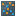#### Versions

You are viewing the RapidMiner Studio documentation for version 8.2 - Check here for latest version

#Cluster Model Visualizer (Model Simulator)

## Synopsis

This operator uses visualization tools for centroid-based cluster models to capture the essential details of each cluster.

## Description

The visualization tools include the following:

• Overview: shows the size of all found clusters, together with some information about the clusters and their quality.
• Heat map:: displays a decision tree describing the main difference between the clusters.
• Centroid Chart: shows the values for the cluster centroids in a parallel chart.
• Centroid table: shows the values for the cluster centroids in a table.
• Scatter plot: with a choice of cluster, displays a scatter plot in terms of the two most important Attributes.

## Input

•model (Centroid Cluster Model)

This input port expects a centroid-based cluster model.

•clustered data (Data Table)

This input port expects a clustered ExampleSet which is the output of the cluster model building process.

## Output

•visualization output

This output port provides visualization tools to help understand clusters.

•model output (Centroid Cluster Model)

The input model is passed without changing to the output through this port.

## Tutorial Processes

### Visualizing Cluster for Iris

This process creates a cluster model on the Iris data set. We use the very common k-Means clustering algorithm with k=3, i.e. we want to find three clusters in the data. The cluster model is then delivered together with the clustered data to the Cluster Model Visualization operator, which creates the visualizations.

Examining the output from each of the visualization tools, we find the following: Overview: Cluster 1 is the biggest cluster with 61 items. Heat map: Cluster 0 has on average much higher values for a1, a3, and a4. The cluster tree, centroid chart, centroid table, and scatter plot show the same results in a different form.